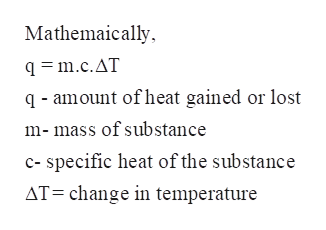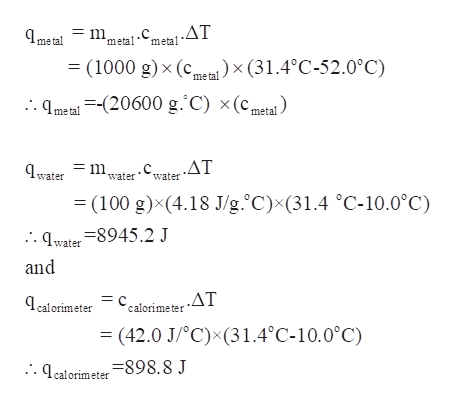# A 1.00kg piece of metal at 52.0C is placed into 100. g of water at 10.0C. The final temperature of the water is 31.4C. Assuming a coffee cup calorimeter heat capacity of 42.0 J/C, calculate the specific heat of the metal. The specific heat of water is 4.18 J.gC.

Question

A 1.00kg piece of metal at 52.0C is placed into 100. g of water at 10.0C. The final temperature of the water is 31.4C. Assuming a coffee cup calorimeter heat capacity of 42.0 J/C, calculate the specific heat of the metal. The specific heat of water is 4.18 J.gC.

check_circle

Step 1

A 1.00 kg piece of metal at 52.0°C is placed into 100. g of water at 10.0C. The final temperature of the water is 31.4°C. Assuming a coffee cup calorimeter heat capacity of 42.0 J/°C, the specific heat of the metal is to be calculated. The specific heat of water is 4.18 J/g°C.

Step 2

The specific heat of any substance is the amount of heat required by unit mass of substance to raise its temperature b 1°C.help_outlineImage TranscriptioncloseMathemaically q =m.c.AT q-amount of heat gained or lost m-mass of substance c- specific heat of the substance AT=change in temperature fullscreen
Step 3

As the temperature of metal is decreasing, it gives off its heat to the calorimeter containing water.

Therefore, qmetal = -(qwater +qcalorim...help_outlineImage Transcriptionclosemetal-Cmeta!.AT (1000 g) x (cmx (31.4°C-52.0°C) metal . qmeta =-(20600 g. C) x(cmetal =mwater ,.ΔΤ water = (100 g)x(4.18 J/g.°C)x(31.4 °C-10.0°C) .water qwater 8945.2 J and , .ΔΤ ealorimeter calorime ter = (42.0 J/°C)x(31.4°C-10.0°C) .qcalorimeter-898. 8 J fullscreen

### Want to see the full answer?

See Solution

#### Want to see this answer and more?

Solutions are written by subject experts who are available 24/7. Questions are typically answered within 1 hour.*

See Solution
*Response times may vary by subject and question.
Tagged in

### Chemical Thermodynamics